# Using Pivot Table Calculated FieldsPivot table are a great way to analyses the data for an excel user. Most of the times, the pivot tables are produced using existing fields (or variables). These fields are added to various parts of pivot table to get meaningful analysis of the data.

But at some times, these simple calculations are not sufficient to give us a specific analysis we are looking for. We might be interested in some ratios or percentages that we usually don’t get directly from such pivot tables. In this case we revert to a pivot table tool that is called “Calculated Field”.

A calculated field is a field or variable that is created by using one or more existing fields. We actually manipulate one or more fields to get a new one that is more of our use and then we do analysis using that field.

In this post, we will take up an example and see how we can create a perform analysis using “Calculated Fields”.

Example:

We have a sample database – its database showing sales of a stationary shop that sales through three channels:

• Online Channel

• Through Tuck shop in the community school

• Through a retail shop near the school

The shop has variety of items related to stationary. The data looks like following: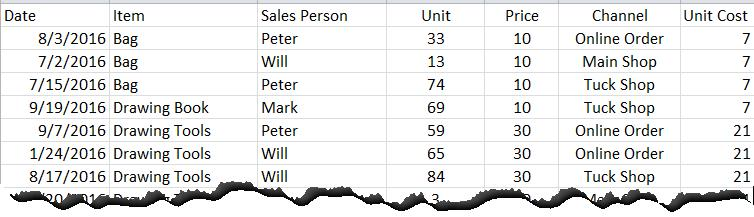The fields in this table are Date, Item, Sales Person, Unit, Price, Channel and Unit Cost.

A typical pivot table can list various scenarios like sales breakup for each sales person, sales breakup for each item, breakup of sales with and item and so on. But what if you want to calculate Total Sales that is not provided in the data itself? In this case we need to create a “calculate field”.

Creating a Calculated field:

For Excel 2010, calculated fields can be accessed when you have created the Pivot Table. Once you have created one, point your cursor the pivot table, this will enable the pivot table options.

From Pivot Table options tab, select: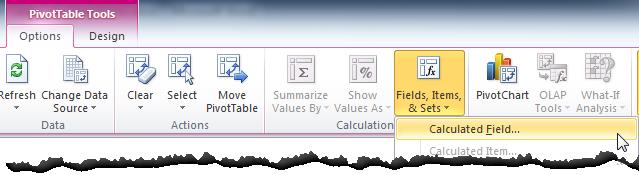When you select the “calculated field” the following dialogue box will prompt. We need to select the field “Price”, put an asterisk that represents the multiplication sign and then select the second field “Unit” and then press Ok.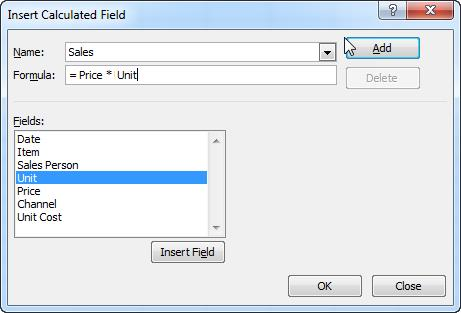The new calculated field will be added to the field list and as you drag the field the “value” section of the table, the following table will be displayed: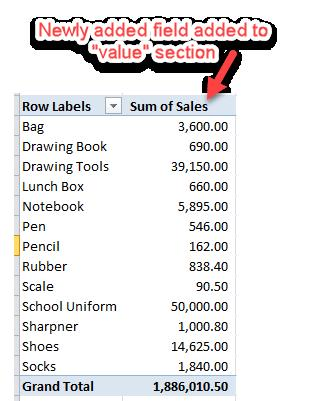In this way you have created a new variable or field is crated that was not part of the initial database.

Example # 2

You want to create a scenario where you want to see channel wise profit margin. But there is no as such field that shows “Profit”. We need to calculate the pivot table calculated field that shows the difference of “Price” and “Unit Cost” multiplied by sales unit to give the result.

We workout following calculated field: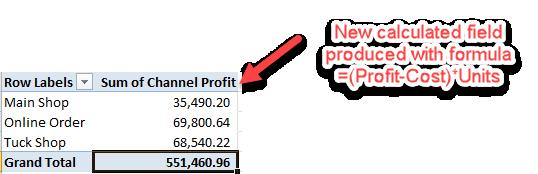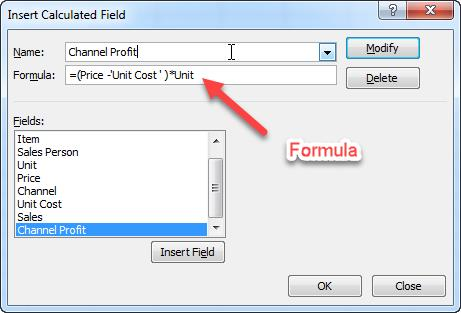Conclusion:

“Calculated Field” is powerful means of getting desired result. You can avoid your database to be bulky by creating meaningful fields and improve your analysis with it. Please find attached worksheet to see how it practically works.